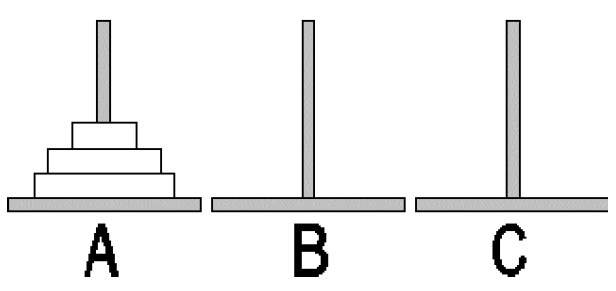# 2016-2017 ACM Central Region of Russia Quarterfinal Programming Contest B. Hanoi tower

## 原题

Gym 101243B

It has become a good tradition to solve the “Hanoi tower” puzzle at programming contests in Rybinsk. We will review the rules briefly. There are 3 rods marked A, B, C. Initially, N disks of different diameter are placed on rod A: the smallest disk is at the top, the disks below it are
ordered by diameter, in increasing order. Rods B and C are empty yet. The goal is to move all disks from rod A to rod B, using rod C as auxiliary.At each step you can take the uppermost disk from any rod and then put it either on
an empty rod or on a rod where the diameter of the uppermost disk is greater than the
diameter of the disk taken.
Many books on programming give a recursive solution of this task. Here is a sample
procedure in Pascal.

Procedure Hanoi (X, Y, Z: char; N: integer);
Begin
If N>0 then
Begin
Hanoi (X, Z, Y, N-1);
Writeln(‘Disk ’, N, ‘ from ‘, X, ‘ to ‘, Y);
Hanoi (Z, Y, X, N-1)
End
End;


Your task is to write a program that will determine the number of the move, after
which the disks for the first time after the start of the game are distributed equally
between the rods.

Input

The input file contains an integer N – the number of disks.

Output

The output file must contain an integer number – the number of the move, after
which the disks for the first time after the start of the game are distributed equally
between the rods.

Limitations

1 <= N <=300 , N mod 3 = 0

Examples

Input Output
3 6
6 9

## 题解

Hanoi tower通式为 2^n-1

#include <iostream>
#include <algorithm>
#include <cstdio>
#include <cstdlib>
#include <cmath>

using namespace std;

/* 用于test2记录移动情况 */
int k = {0};
double ans1 = 0;
int ans2 = 0;

double hanoi(int n)
{
return round(pow(2, n)-1);
}

int hanoiTest1(int n)    //得到hanoi的通式
{
if (n < 1) return 0;
int a = hanoiTest1(n-1);
a ++;
a += hanoiTest1(n-1);
return a;
}

int hanoiTest2m(int a, int b)
{
if (k == k && k == k) return 0;
k[a]--; k[b]++;
ans2++;
return 1;
}

void hanoiTest2(int a, int b, int c, int n)
{   /*模拟移动 发现奇数时候不太对劲*/
if (n <= 0) return;
hanoiTest2(a, c, b, n-1);
hanoiTest2m(a, b);
hanoiTest2(c, b, a, n-1);
}

int main()
{
//freopen("input.txt", "r", stdin);
//freopen("output.txt", "w", stdout);

for (int i = 3; i <= 60; i+=6) {
k = i;k = 0; k = 0;
ans2 = 0;
hanoiTest2(0, 1, 2, i);
/* 最初的想法 先从a移动i*(2/3)-1个到b 再移动一个到c 最后将i/3-1个从b移动到c */
if (i % 2 == 0) ans1 = hanoi(i/3*2-1) + 1 + hanoi(i/3-1);
/* 如果奇数需要移动第二步两次(规律如此 实际移动方法并不) */
else ans1 = hanoi(i/3*2-1) + 1 + hanoi(i/3-1) * 2;
printf("%d %.0lf %d\n", i, ans1 , ans2);
}

return 0;
}

import java.io.*;
import java.math.*;
import java.util.*;

public class Main {
public static void main(String[] args) {
try {
/*
FileInputStream inFile = new FileInputStream("input.txt");
PrintStream outStream = new PrintStream(new FileOutputStream("output.txt"));

System.setIn(inFile);
System.setOut(outStream);
*/
Scanner sc = new Scanner(System.in);
int a = sc.nextInt();

BigInteger ans = hanoi(a / 3 * 2 - 1);
ans = ans.add(hanoi(a / 3 - 1));
if (a % 2 == 1) ans = ans.add(hanoi(a / 3 - 1));

System.out.println(ans.toString());
sc.close();
} catch (Exception e) {
}
}

public static BigInteger hanoi(int n) {
BigInteger ans = new BigInteger("2");
ans = ans.pow(n);
return ans.subtract(BigInteger.ONE);
}
}

posted @ 2021-05-23 16:19  八衛門狸  阅读(32)  评论(0编辑  收藏  举报